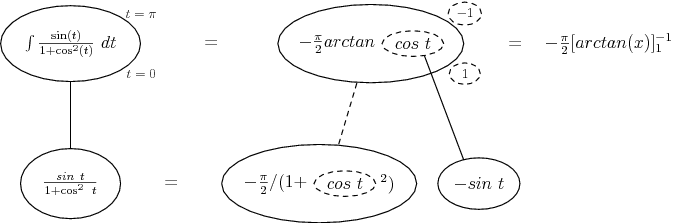# Thread: Definite Integration.

1. ## Definite Integration.

Evaluate

$\displaystyle I = \int_{0}^{\pi} \frac{x sin x}{1+cos^2 x} dx$

2. Hello,Originally Posted by varunnayuduEvaluate

$\displaystyle I = \int_{0}^{\pi} \frac{x sin x}{1+cos^2 x} dx$
Let $\displaystyle t=\pi-x$ :

$\displaystyle I=-\int_\pi^0 \frac{(\pi-t) \sin(\pi-t)}{1+\cos^2(\pi-t)} ~ dt=\int_0^\pi \frac{(\pi-t) \sin(\pi-t)}{1+\cos^2(\pi-t)} ~ dt$

But $\displaystyle \sin(\pi-t)=\sin(t)$ and $\displaystyle \cos(\pi-t)=-\cos(t)$
Hence :

$\displaystyle I=\int_0^\pi \frac{(\pi-t) \sin(t)}{1+\cos^2(t)}~dt$

$\displaystyle I=\pi \int_0^\pi \frac{\sin(t)}{1+\cos^2(t)} ~dt-\underbrace{\int_0^\pi \frac{t \sin(t)}{1+\cos^2(t)} ~ dt}_{=I}$

$\displaystyle 2I=\pi \int_0^\pi \frac{\sin(t)}{1+\cos^2(t)} ~ dt$

$\displaystyle I=\frac{\pi}{2} \int_0^\pi \frac{\sin(t)}{1+\cos^2(t)} ~ dt$

Now substitute $\displaystyle \varphi=\cos(t)$ and it'll be easy.

3. ## hw did u do that

hw did u get that

4.Originally Posted by zorrohw did u get that
You will need to be more specific. What part of Moo's reply don't you understand?

5. ## Please tell me if its correct.Originally Posted by MooHello,

Let $\displaystyle t=\pi-x$ :

$\displaystyle I=-\int_\pi^0 \frac{(\pi-t) \sin(\pi-t)}{1+\cos^2(\pi-t)} ~ dt=\int_0^\pi \frac{(\pi-t) \sin(\pi-t)}{1+\cos^2(\pi-t)} ~ dt$

But $\displaystyle \sin(\pi-t)=\sin(t)$ and $\displaystyle \cos(\pi-t)=-\cos(t)$
Hence :

$\displaystyle I=\int_0^\pi \frac{(\pi-t) \sin(t)}{1+\cos^2(t)}~dt$

$\displaystyle I=\pi \int_0^\pi \frac{\sin(t)}{1+\cos^2(t)} ~dt-\underbrace{\int_0^\pi \frac{t \sin(t)}{1+\cos^2(t)} ~ dt}_{=I}$

$\displaystyle 2I=\pi \int_0^\pi \frac{\sin(t)}{1+\cos^2(t)} ~ dt$

$\displaystyle I=\frac{\pi}{2} \int_0^\pi \frac{\sin(t)}{1+\cos^2(t)} ~ dt$

Now substitute $\displaystyle \varphi=\cos(t)$ and it'll be easy.
--------------------------------------------------------------------

$\displaystyle cos(t) = \varphi$
therefore
$\displaystyle - sin t dt= d\varphi$

$\displaystyle - \frac{\pi}{2} \int_0^\pi \frac{1}{1+\varphi^2}d\varphi$

what to do next ................6.Originally Posted by zorro--------------------------------------------------------------------

$\displaystyle cos(t) = \varphi$
therefore
$\displaystyle - sin t dt= d\varphi$

$\displaystyle - \frac{\pi}{2} \int_0^\pi \frac{1}{1+\varphi^2}d\varphi$

what to do next ................$\displaystyle \int \frac{1}{1+\varphi^2} \, d\varphi$ is a standard form and is equal to $\displaystyle \tan^{-1} \varphi$ (I have omitted the arbitrary cnostant of integration).

7.Originally Posted by mr fantastic$\displaystyle \int \frac{1}{1+\varphi^2} \, d\varphi$ is a standard form and is equal to $\displaystyle \tan^{-1} \varphi$ (I have omitted the arbitrary cnostant of integration).

--------------------------------

= -$\displaystyle \frac{\pi}{2} (tan^{-1} \pi - tan^{-1}0)$

= - $\displaystyle \frac{\pi}{2} (0-0)$

= - $\displaystyle \frac{\pi}{2} .0$

= $\displaystyle 0$

is this right .........8. You forgot to change the limits.

9.The straight dashed line diff's / anti-diff's with respect to the dashed balloon expression.

Don't integrate - balloontegrate! http://www.ballooncalculus.org/examples]

10. ## hw did t conert to piOriginally Posted by tom@ballooncalculusThe straight dashed line diff's / anti-diff's with respect to the dashed balloon expression.

Don't integrate - balloontegrate! http://www.ballooncalculus.org/examples]

-------------------------------------------------------------------

Here hw did t=$\displaystyle \pi$ turn into 1 and -1.

11.Originally Posted by zorro-------------------------------------------------------------------

Here hw did t=$\displaystyle \pi$ turn into 1 and -1.
Recall that there was a change of variable: $\displaystyle \varphi = \cos t$.

$\displaystyle t = 0 \Rightarrow \varphi = \cos 0 = 1$.

$\displaystyle t = \pi \Rightarrow \varphi = \cos \pi = -1$.

12.Originally Posted by zorro-------------------------------------------------------------------

Here hw did t=$\displaystyle \pi$ turn into 1 and -1.
they are the limits for the new variable

#### Search Tags

definite, integration